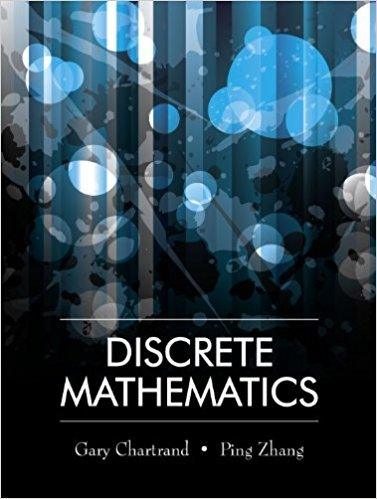×
×

# Solutions for Chapter 3.4: Proof by Cases## Full solutions for Discrete Mathematics | 1st Edition

ISBN: 9781577667308Solutions for Chapter 3.4: Proof by Cases

Solutions for Chapter 3.4
4 5 0 320 Reviews
17
1
##### ISBN: 9781577667308

Discrete Mathematics was written by and is associated to the ISBN: 9781577667308. Chapter 3.4: Proof by Cases includes 16 full step-by-step solutions. This expansive textbook survival guide covers the following chapters and their solutions. Since 16 problems in chapter 3.4: Proof by Cases have been answered, more than 13779 students have viewed full step-by-step solutions from this chapter. This textbook survival guide was created for the textbook: Discrete Mathematics, edition: 1.

Key Math Terms and definitions covered in this textbook
• Affine transformation

Tv = Av + Vo = linear transformation plus shift.

• Back substitution.

Upper triangular systems are solved in reverse order Xn to Xl.

• Complete solution x = x p + Xn to Ax = b.

(Particular x p) + (x n in nullspace).

• Eigenvalue A and eigenvector x.

Ax = AX with x#-O so det(A - AI) = o.

• Free variable Xi.

Column i has no pivot in elimination. We can give the n - r free variables any values, then Ax = b determines the r pivot variables (if solvable!).

• Full column rank r = n.

Independent columns, N(A) = {O}, no free variables.

• Gauss-Jordan method.

Invert A by row operations on [A I] to reach [I A-I].

• Identity matrix I (or In).

Diagonal entries = 1, off-diagonal entries = 0.

• Krylov subspace Kj(A, b).

The subspace spanned by b, Ab, ... , Aj-Ib. Numerical methods approximate A -I b by x j with residual b - Ax j in this subspace. A good basis for K j requires only multiplication by A at each step.

• Linearly dependent VI, ... , Vn.

A combination other than all Ci = 0 gives L Ci Vi = O.

• Minimal polynomial of A.

The lowest degree polynomial with meA) = zero matrix. This is peA) = det(A - AI) if no eigenvalues are repeated; always meA) divides peA).

• Nilpotent matrix N.

Some power of N is the zero matrix, N k = o. The only eigenvalue is A = 0 (repeated n times). Examples: triangular matrices with zero diagonal.

• Nullspace matrix N.

The columns of N are the n - r special solutions to As = O.

• Orthonormal vectors q 1 , ... , q n·

Dot products are q T q j = 0 if i =1= j and q T q i = 1. The matrix Q with these orthonormal columns has Q T Q = I. If m = n then Q T = Q -1 and q 1 ' ... , q n is an orthonormal basis for Rn : every v = L (v T q j )q j •

• Partial pivoting.

In each column, choose the largest available pivot to control roundoff; all multipliers have leij I < 1. See condition number.

• Row space C (AT) = all combinations of rows of A.

Column vectors by convention.

• Symmetric factorizations A = LDLT and A = QAQT.

Signs in A = signs in D.

• Vandermonde matrix V.

V c = b gives coefficients of p(x) = Co + ... + Cn_IXn- 1 with P(Xi) = bi. Vij = (Xi)j-I and det V = product of (Xk - Xi) for k > i.

• Vector space V.

Set of vectors such that all combinations cv + d w remain within V. Eight required rules are given in Section 3.1 for scalars c, d and vectors v, w.

• Vector v in Rn.

Sequence of n real numbers v = (VI, ... , Vn) = point in Rn.

×